zoukankan      html  css  js  c++  java
• # 预测功率和电流之间的关系

通常机器学习的开发流程包括：数据收集---数据清洗与转换---模型训练---模型测试---模型部署与整合

下面，通过一个例子进行完整的机器学习开发流程的学习。

工程中需要的库：

```from sklearn.model_selection import train_test_split
from sklearn.linear_model import LinearRegression
from sklearn.preprocessing import StandardScaler
from sklearn.externals import joblib

import numpy as np
import matplotlib as mpl
import matplotlib.pyplot as plt
import pandas as pd
from pandas import DataFrame
```

（1）首先数据的收集，获取数据：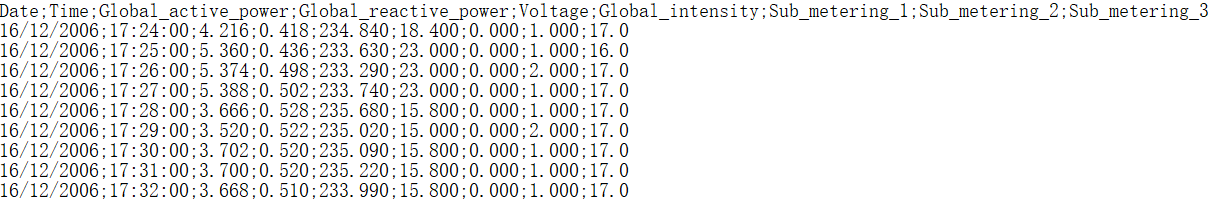如上，可以看到，我们获得需要获取的是Global_active_power（有功功率）、Global_reactive_power（无功功率）和Global_intensity（电流）之间的关系。

```path = 'household_power_consumption_1000.txt'
df = pd.read_csv(path,sep = ';',low_memory = False)

print(df.info())
```

（2）然后进入数据清洗阶段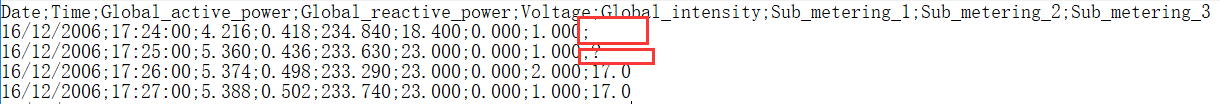如上， 如果存在空值或异常值的情况，我们可以在这个阶段进行处理。

```new_df = df.replace('?',np.nan)
datas = new_df.dropna(axis = 0,how = 'any')
print(datas.describe().T)
```

如上，可以看到，我们对于异常值和空值存在的情况下，直接删掉了该样本。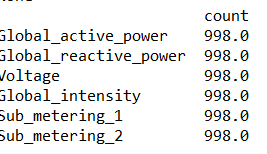可以看到，1000个样本只剩下了998个了。

然后继续进行特征工程处理：

```#提取出相关数据
X = datas.iloc[:,2:4]
Y = datas['Global_intensity']
#划分训练集和测试集
X_train,X_test,Y_train,Y_test = train_test_split(X,Y,test_size = 0.2,random_state = 0)
#标准化处理
ss = StandardScaler()
X_train = ss.fit_transform(X_train)
X_test = ss.transform(X_test)
```

Tips:

• random_state：是随机数的种子。随机数种子：其实就是该组随机数的编号，在需要重复试验的时候，保证得到一组一样的随机数。比如你每次都填1其他参数一样的情况下你得到的随机数组是一样的。但填0或不填，每次都会不一样。
• StandardScaler:标准化需要计算特征的均值和标准差，公式表达为：。至于为什么要做标准化，https://zhuanlan.zhihu.com/p/24839177

（3）模型训练

```lr = LinearRegression()
lr.fit(X_train,Y_train)
```

如上，我们可以通过sklearn封装好的简单的语句完成模型的训练。

（4）模型预测

```y_predict = lr.predict(X_test)
print("训练：",lr.score(X_train,Y_train))#
print("测试：",lr.score(X_test,Y_test))

mse = np.average((y_predict-Y_test)**2)
rmse = np.sqrt(mse)
print(rmse)
```

如上：score是一个评分函数，即R2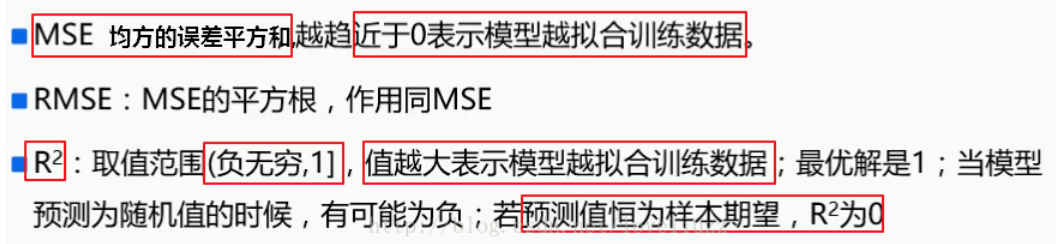数据的可视化：

```## 设置字符集，防止中文乱码
mpl.rcParams['font.sans-serif']=[u'simHei']
mpl.rcParams['axes.unicode_minus']=False
t = np.arange(len(X_test))
plt.figure()
plt.plot(t,Y_test,'r-',label = u'真实值')
plt.plot(t,y_predict,'b-',label = u'预测值')
plt.legend(loc = 'upper right')
plt.title(u'线性回归预测功率与电流之间的关系')
plt.grid(b = True)
plt.show()
```

对于Anaconda2和3同时装的情况下，执行3可以采用下面命令：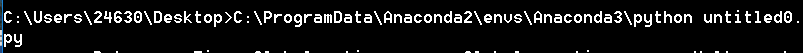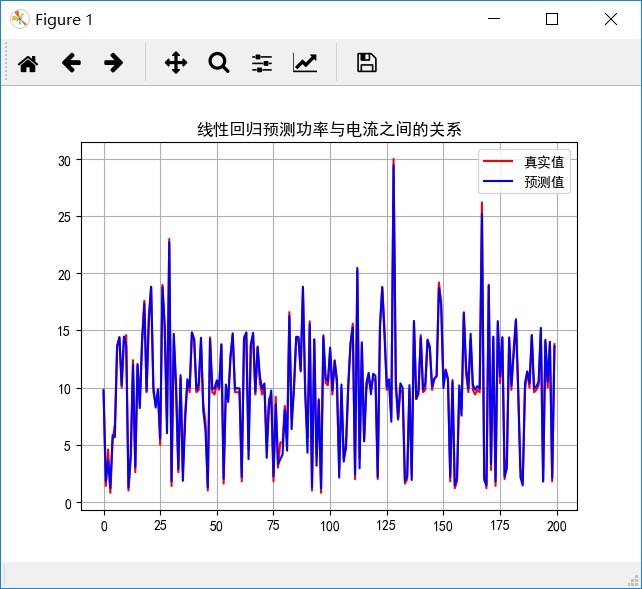（5）模型部署

```joblib.dump(lr,"data_lr.model")

```

PS：当然这里的模型选择也可以选择其他算法，譬如SVR

模型构建步骤改为：

```rbf=svm.SVR(kernel='rbf',C=1, )
rbf.fit(X_train,Y_train)
```

当然，这里的话就需要调参了

• 相关阅读:
paip.调用GUI接口.
paip.按键替换映射总结
paip.IIS 7.5 应用程序池,应用程序以及虚拟目录的存储位置
paip.android 手机输入法制造大法
paip.设置鼠标灵敏度API
paip.系统无法在消息文件中为 Application 找到消息号为 0x2350 的消息文本。服务器存储空间不足，无法处理此命令
paip.DEVSUIT WEB .NET ASPX网站打开慢的原因
paip.动画透明淡入淡出窗口之重绘性能
PAIP。JS调用DLL的解决方案
paip.输入法编程四级非常用汉字汉字1000个
• 原文地址：https://www.cnblogs.com/pinking/p/8970983.html